Share

# Writing Chemical Formulae - Formulae of Simple Compounds

#### notes

The chemical formula of a compound is a symbolic representation of its composition. The chemical formulae of  different compounds can be written easily. For thisexercise, we need to learn the symbols and combining capacity of the  lements.The combining power (or capacity) of an element is known as its valency. Valency can be used to find out how the atoms of an element will combine with the atom(s) of another element to form a chemical compound. The valency  of the atom of an element can be thought of as hands or arms of that atom.

Human beings have two arms and an octopus has eight. If one octopus has to catch hold of a few people in such a manner that all the eight arms of the octopus and both arms of all the humans are locked, how many humans do you  hink the octopus can hold? Represent the octopus with O and humans with H. Can you write a formula for this combination? Do you get OH4 as the formula? The subscript 4 indicates the number of humans held by the octopus.

FORMULAE OF SIMPLE COMPOUNDS :

The simplest compounds, which are made up of two different elements are called binary compounds. Valencies of some ions are given in Table 3.6. You can use these to write formulae for compounds. While writing the chemical  formulae for compounds, we write the constituent elements and their valencies as shown below. Then we must crossover the valencies of the combining atoms.

Examples :

1. Formula of hydrogen chloride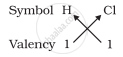Formula of the compound would be HCl

2. Formula of hydrogen sulphide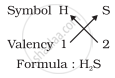3. Formula of carbon tetrachloride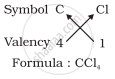For magnesium chloride, we write the symbol of cation (Mg2+) first followed by the symbol of anion (Cl- ). Then their charges are criss-crossed to get the formula.

4. Formula of magnesium chloride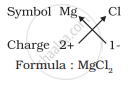Thus, in magnesium chloride, there are two chloride ions (Cl- ) for each magnesium ion (Mg2+). The positive and negative charges must balance each other and the overall structure must be neutral. Note that in the formula, the  charges on the ions are not indicated.

(a) Formula for aluminium oxide: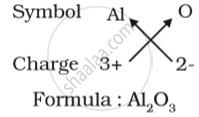(b) Formula for calcium oxide: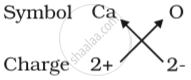Here the valencies of the two elements are the same. you may arrive at the formula Ca2O2 But we simplify the formula as CaO.

(c) Formula of sodium nitrate: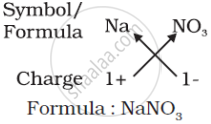(d) Formula of calcium hydroxide: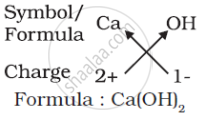Note that the formula of calcium hydroxide is Ca(OH)2 and not CaOH2. We use brackets when we have two or more of the same ions in the formula. Here, the bracket around OH with a subscript 2 indicates that there are two hydroxyl (OH) groups joined to one calcium atom. In other words, there are two atoms each of oxygen and hydrogen in calcium hydroxide.

### Shaalaa.com

Atoms and Molecules (Chemical Formula Rules) [00:13:09]
S
series 1
0%

S